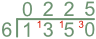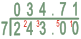Short Division

# Short Division

Basic, GCSE(F),

Division splits one number into a number of smaller, equal parts.  The number carrying out the dividing is called the divisor. Short Division is the division of one number by a single digit.

Place the number to be divided under a bus stop, with the divisor to the left of the vertical line. the answer will be written above the horizontal line.

Division takes place one digit at a time, working from left to right. The number of complete times that the divisor divides into each digit is written above the digit, and the remainder carried forward (as a multiple of 10) to the next number.

At the end of a division, there may be a remainder:

• the remainder may be shown as an integer;
• the remainder may be shown as a fraction (remainder/divisor);
• add places (zeroes) after the decimal point for a decimal answer.

## Examples

1. A company is sending holiday brochures to its six travel shops.  If 1350 brochures are equally distributed to each shop, how many brochures will each shop receive?

This is a division: divide 1350 by 6.6 into 1: 0, remainder 1 (changes the next number from 3 to 13);
6 into 13: 2 (place above the 3), remainder 1 (the next number becomes 15);
6 into 15: 2 (place above the 5), remainder 3 (the next number becomes 30);
6 into 30: 5 (place above the 0), no remainder.

2. Divide 243 by 7. Show the result of the division a) as a remainder; b) as a fraction, and c) as a decimal to 1 decimal place.

Answer: a) 34 remainder 5; b) 34frac(5)(7) ; c) 34.7

Show the calculation with a remainder:

a) As a remainder: 243 ÷ 7b) As a fraction, show the remainder over the divisor (frac(5)(7)) to give an answer of 34frac(5)(7).

c) Add a decimal point and create decimal places using zeroes. The number of decimal places must be one more than the accuracy required. Check also for rounding. Divide 243.00 by 7.